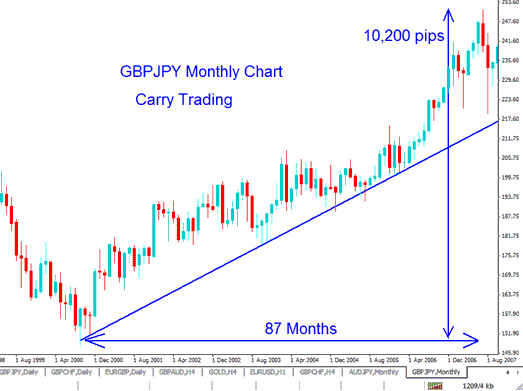# Forex Carry Trading: Long Term Strategy - What is it

Carry Strategy is one of the long term investment strategy used. it is the use of interest rates differences to profit from currency trades.Example of a This Strategy on GBPJPY Pair

A carry trade is when a currency with a lower rate is sold to purchase a one that pays a higher rate. The difference between the two currencies is called the interest rate differential.

When traders select a currency pair, they will choose a the pair that will pay them a higher rate, buy that currency and sell the one that pays lower and make a profit from this difference.

Since this strategy uses two currency pairs, where a one simultaneously buys one currency and sell the other, the one will buy the one with higher interest and sell the one with a lower interest rate.

For example in the above GBPJPY chart there was a perfect carry trading setup, this was because the GBP was paying higher interest of about 5% and the JPY one would only pay 0.5%.

The difference was 4.5%, this difference propelled the GBPJPY upwards for a total of 87 months about 7 and half years. The currency pair moved a total of 10,200 pips which is equivalent to 102 cents, 100 pips = 1 cent.

The reason for the upward trend is that it was profitable to hold the GBP compared to JPY as it paid a difference of 4.5% interest to the investor on the other hand selling GBP against JPY, you as the investor will pay the 4.5% from your own money.

After this carry trade, there was carry unwinding after 2008 recession where GBP economy reduced the interest rate on GBP to about just 0.5% to spur economic growth (and spur quantitative easing) an interest rate level almost the same as that of JPY, meaning that there was no much difference or profit to be obtained by buying the GBP against the JPY. This is known as Carry Unwinding. Some investors may refer to it as negative carry.

## Carry Example: Using AUDJPY

If the AUD has an interest of 5 %

And

The JPY has interest of 0.5 %

Then we can use this difference to carry the AUDJPY

## The question is how to implement this strategy?

First we shall buy the AUDJPY

This will mean that we now have bought AUD and simultaneously sold JPY.

Because we have bought AUD, we shall be paid the a rate of 5%.

At the same time we have sold JPY, we shall pay an a rate of 0.5%.NB: If you buy a currency you get paid the interest, when you sell a currency you pay interest.

For AUD we get paid 5% per year

For JPY we pay 0.5% per year

Since we get paid 5% and we pay 0.5% the difference (profit) is 4.5%.

This difference is what is called the carry trade - Exploiting interest rates difference to make a profit on top of the profit that you get from pips. This is like double profit.

With this strategy we always buy the higher interest rate currency and sell the lower rates one for a positive yield. If your trade is in the opposite direction then you will be the one paying the interest. Using the this method is a great way to earn excellent rates of return on your investment, profit from the rates as well as the capital gain from the transaction also.

A carry trade provides the opportunity to make high returns in the forex market especially when leverage is used. In the example below we show you how Forex leverage increases the returns from a this type of investing.

## Examples

Using the AUDJPY example above:

If you have \$50,000 and buy the AUDJPY For 1 year you will get 4.5%

Interest for that year.

4.5% of \$50,000= \$2,250

This Profit is not so much for 50,000 dollars, but when you add Forex leverage the profits will be magnified.

Example 1: Leverage 5:1

Now what if we use Leverage of 5:1

You have \$50,000 add the leverage= 50,000*5= \$250,000

Now if you have 250,000 then 4.5% of this money=

4.5% of \$250,000= \$11,250

Profit = \$11,250

Capital = \$50,000

return = 11250/50000= 22.5%

Example 2: Leverage 10:1

Now what if we use Leverage of 10:1

You have \$50,000 add the leverage= 50,000*10= \$500,000

Now if you have 500,000 then 4.5% of this money=

4.5% of \$500,000= \$22,500

Profit = \$22,500

Capital = \$50,000

return = 22500/50000= 45%

When you leverage your money when placing the trades the profit from your money will also then be multiplied. Its best to keep leverage below 5:1 because these is a long term strategy, and buy when price is close to upward trend line where there is a good risk: reward ratio.

From the above example, the profit is magnified by the use of leverage, by using a leverage of 10% the above example transaction would have yielded a 45% per year. This is also not including the profits that would have been made from the capital gains i.e. pips profit. to learn the various factors to consider read this topic: Factors of Carry.

### Popular Currencies for this strategy

The most Popular Currencies are the Yen Currency Crosses, These are:

GBPJPY - Great Britain Pound vs Japanese Yen

AUDJPY - Australian Dollar vs Japanese Yen

NZDJPY - New Zealand Dollar vs Japanese Yen